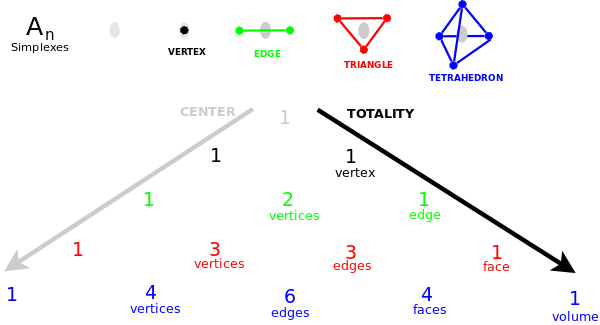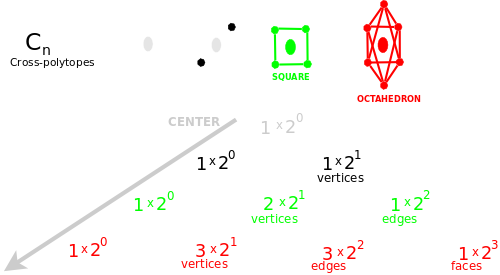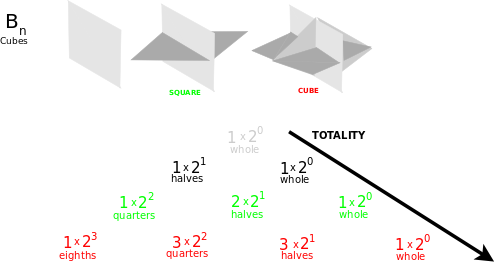• ms@ms.lt
• +370 607 27 665
• My work is in the Public Domain for all to share freely.

Introduction E9F5FC

Understandable FFFFFF

Questions FFFFC0

Notes EEEEEE

Software

I am deriving the answer from the 4 different kinds of symmetries inherent in choice. These yield the following four interpretations of the binomial theorem in terms of polytopes:

• {$\prod_{i}(\varnothing + \leftrightarrow_{i})$} Simplexes.
• {$\prod_{i}(\varnothing + (\leftarrow_{i} + \rightarrow_{i}))$} Cross-polytopes.
• {$\prod_{i}((\leftarrow_{i} + \rightarrow_{i})+\leftrightarrow_{i})$} Cubes.
• {$\prod_{i}(\leftarrow_{i} + \rightarrow_{i})$} Cube slices.

Note that swapping {$\leftarrow_{i}$} and {$\rightarrow_{i}$} maps {$\leftrightarrow_{i}$} to itself. This is why the Weyl group has order {$2^n n!$} and not, say, {$3^n n!$}. Thus {$\leftrightarrow_{i}$} is similar to {$\varnothing$} but it behaves as a pseudocenter (much like a pseudoscalar, the highest grade element in a Clifford algebra). A whole is a pseudocenter in that it is only defined with regard to a set of known dimensions whereas a center is defined with regard to all possible dimensions. The known dimensions are the ones which an index runs over.

How to relate (-n,...,-2,-1) with (1,2,...,n)? along the diagonal of a matrix (the Cartan subalgebra). So that they maintain a duality. What are the ways of supporting the duality in a counting process?

I need to relate these to the ways of linking up two sequences. Linking ...XYZ and ...ABC can have the following solutions:

• XYZABC (folding)
• XYZ0ABC (linking externally - odd!)
• XY0BC where 0=Z=A (linking internally - even!)

and this lastmost statement is an equivalence, thus this is a context for defining equivalence.

Dorovės atžvilgiu, tai susitarimo galimybės. Vidinės ir išorinės?

In geometry, rotating by 90 degrees makes for independence. So the root systems impose this on the algebra.

If I make three choices, then a fourth choice "left" makes a difference; whereas a fourth choice of not choosing makes no difference - the center of a triangle stays the same no matter how many dimensions you add.

Interpret (A-B)^N.

Conjugacy classes counted by expanding multinomial theorem where certain letters (choices) are set the same.

Lie algebra linking - is given by placing a cube on its corner and projecting its vertices onto the plane, yielding A2.

Note that ← and → add to yield paths. They are within the same system. Whereas \ast and \ast are in different systems.

Crucial fact is its about the inherent geometry in sequential choices. (Choices made one-by-one as opposed to being made all at once.)

Extrinsic vs. intrinsic.

Slack lets you turn around.

• Coordinate system - broken up relations, qualities (counting vertices) - existence is internal - dimensions are not ordered - commutative.
• Simplex - existence is external - relations of things with regard to each other (counting subsets)(ordered choices, vertices) - noncommutative.

A coordinate system (sliced cube) has each vertex face in two directions (left and right). But in a simplex the vertices face in only one direction (outside not inside). (Compare with hyperoctahedral group in terms of shuffling cards with two sides.)

Explicit choice "thing" vs. "no-thing" ("choose" vs. "don't choice") choices yield a syntactically asymmetric outcome "everything" vs. "nothing", whereas implicit choice "this" vs. "that" yields a syntactically symmetric outcome. (all "this" and all "that" is the same).

Commutative or noncommutative?

The choice itself is different ... or what is chosen (this or that)?

Observer and observed. An observer waits for the answer. The observed doesn't wait - it has no concept.

Entropy. Reversibility of time (intrinsic geometry) vs. asymmetry of time (extrinsic geometry). Grace requires "extra" states. With justice there are no extra states.

Note that the infinite paths down Pascal's triangle correspond to the real numbers from 0 to 1 in base 2.

How is Pascal's cone - the circular row - related to a finite field? and how it changes with q?

Cube slices have an externally referent system - so when you flip it - you flip the coordinate axes as well - keeping it symmetric. Simplexes have a self-referent system so if you flip it, then you get...?

Walks on trees - binomial theorem

Differentiation (body + edge)^N or (long+short)^N

(left + right) is a repeated experiment, a repeated choice - left is consistent, right is consistent, in each choice - this is needed for doing measurements and experiments

Is it closing a cycle? Or is it turning counting around, as when facing God and turning around to view with him?

Counting Young diagrams: Having empty outer column vs. having nonempty bottom row. How does the q-analogue work?

Indistinguishable boson and distinguishable fermion.

Write up: Conjugation is relabeling.

Dimensions of counting

• Simplex-complex numbers (2 disconnected dimensions)
• Cross-polytopes - reals (1 linked dimension)
• Cubes - reals (1 linked dimension)
• Symplexes - quaternions (4 folded, disconnected dimensions)

Projective linked by adding an extra point - infinity. Affine - internal zero - what would that mean?

• Investigation: Pascal's Cone: Linking together the two sides of Pascal's triangle to form a cone of circles. Do the ways of linking together relate to the 4 polytopes? How does the addition propagation work for cycles? How does it relate to topology where we variously link the sides of a square?
• A polytope built of "centers" (for example, the centers of all the subsimplexes of a simplex). What would it look like?

{$(∅ + \ast)^n$} Simplexes.• The number of ways to choose k elements from an n-element set.
• The subsimplexes of a simplex.

{$(∅ + (← + →))^n$} Cross-polytopes.• Trinomial expansion

{$((← + →)+ \ast)^n$} Cubes.• {$(SHORT + LONG + \ast)^n$} Dividing up a cube.
• {$(BODY + EDGE + \ast)^n$} Differentiating the volume of a cube.

{$(← + →)^n$} Cube slices.

Sources

Related

Q-analogues

M M* = 1 This gives a structural, geometric interpretation for an involution - there is natural symmetry in the form of a matrix - like the root systems - and the point of having orthogonality, orthonormality - a set of combinatorially nice, independent basis elements to work with

• Collect and organize interpretations of the binomial theorem.
• Find q-analogues for each of the four interpretations of the binomial theorem. See how what they count relates to a finite field of characteristic q. Then see what happens to each of them, and their relationship, when q->1.
• How to interpret that two choices (reflections) are linked (noncommutatively) by a hyperplane (at an angle pi/k where RiRj has order k)?
• Four geometries in terms of choices: paths - one-directional, lines - forwards and backwards, angles - left and right, oriented areas - inside and outside. What are the choices with regard to?

Understand

• the relationship between Young tableux and the binomial theorem.
• Schur functions and their role in the representation theory of the general linear group.
• how the binomial theorem relates to:
• homology and the Gauss-Bonnet theorem and Euler's characteristic
• Grassmannians
• the Gaussian binomial coefficients in terms of Coxeter generators
• Q analogue and Decategorification.
• analyze the expression in base 2: {$\sqrt{2} = \sum_{k=0}^{\infty} (2k+1) \binom{2k}{k} 2^{-3k -1}$}
• what Fermat's Last Theorem says: there is no row sum (except the first or second row) for which the sum of two Pascal's triangles can equal that row sum in a third Pascal triangle.
• the relationship between the Young symmetrizer and the special rim hook tableaux
• the combinatorial interpretation of the characters in terms of rim hook tableaux, and their relationship to the Young symmetrizer
• think of convolutions (in functional analysis) as relating left hand side and right hand side of functional composition

Questions

• What do the exceptional Lie groups/algebras mean in terms of expansions of choices? of dimensions 2,4,6,7,8?
• What do the Poincare polynomials mean in terms of choices?
• How can q-analogues be thought of as "counting-in-parallel" and in what sense is that non-deterministic?

Why can't there be brought together three countings (1,2,3...) and (-1,-2,-3...) and (A,B,C...) etc.? or four countings? but only two?

How do cross-polytopes and cubes get distinguished as odd and even?

Compare with my interpretation of Clifford algebras.

Look for connections with Bott periodicity.

In comparing the three levels in my choice model - a comparison is also a choice. And what kind of choice is that? What kind of choice is implicit in the comparison + ?

Relate to linear algebraic group and field with one element and q-analogues

What is the meaning of a root, a transposition (ij) for An, given the choices between * and (1,2,...n)? What does it mean to relate two choices?

How does this all relate to the Axiom of Choice, in particular, indexing over known dimensions or all possible dimensions?

BinomialTheorem

Naujausi pakeitimai

 Puslapis paskutinį kartą pakeistas 2019 vasario 03 d., 22:37